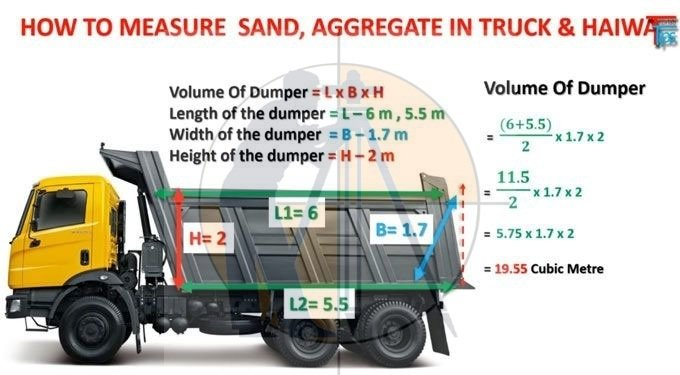Breaking News
Home / QA/QC Engineering / How To Measure Quantity Of Sand In Truck & HAIWA

# How To Measure Quantity Of Sand In Truck & HAIWA

## How To Measure Quantity Of Sand In Truck & HAIWA

This article demonstrates how to measure quantity of sand in truck and HYVA.

Now let us consider the truck in which one side is vertical whereas the opposite side is slanting. Thus, the upper length of the sand in the truck is more than the lower length of the sand. As a result, it becomes confusing when one tries to measure the quantity of sand.Quantity of sand in the upper part of HYVA = 10’9”
Quantity of sand in the lower part of HYVA = 8 ‘6”
Width of the HYVA =6‘

To calculate the height of the HYVA, you need to bring a wooden stick which is not stretchable, but must be rigid. Now insert the wooden stick into the sand and take minimum 3 readings.

The first reading let us consider H1
HI = 5’2”
Second reading let us Consider H2
H2=5’4”
Third Reading let us consider H3
H3=5’

The first step is to find the average of the readings.
H= H1+H2+H3/3

### Basic Estimation And Quantity Of Materials For Brick Wall, U.C.R. Masonry Plastering And Tiles

H1 = 5 ‘2”
=5’+2/12
=5’+0.166
=5.166
H2= 5’4”
=5’+4/12
=5 +0.333
=5.333

Now to find the average by using the formula H= H1+H2+H3/3
=5.166+5.333+5/3
H= 5.166’

The next step is to find the volume of HYVA.

We know V= LXBXH
Quantity of sand in the upper part of HYVA [L1] = 10’9”

Converting to feet
10+9/12
=10+0.75
L1=10.75’
Quantity of sand in the lower part of HYVA[L2] = 8 ‘6”
=8+6/12
L2=8.5

Next step is to find the average length
L= L1+L2/2
=10.75+8.5/2
=9.625’

Length (L)=9.625’
Height(H)=5.166 ft

### How To Calculate The Quantity Of Bricks in 1 m3

Volume in CFT
=9.625’X6’X5.1666
=298.336 cft
Volume in cubic meter
=298.336/35.3147
=8.448 cubic meter
Volume in Brass
=298.336/100
2.983 brass

Volume in Ton = volume in cubic meter x density of sand.

=8.448 x 1600
=13516.8kg
To convert Kg into Ton
=13516.8/1000
13.516 ton

## . Land Surveying & Architects

### THANKS.